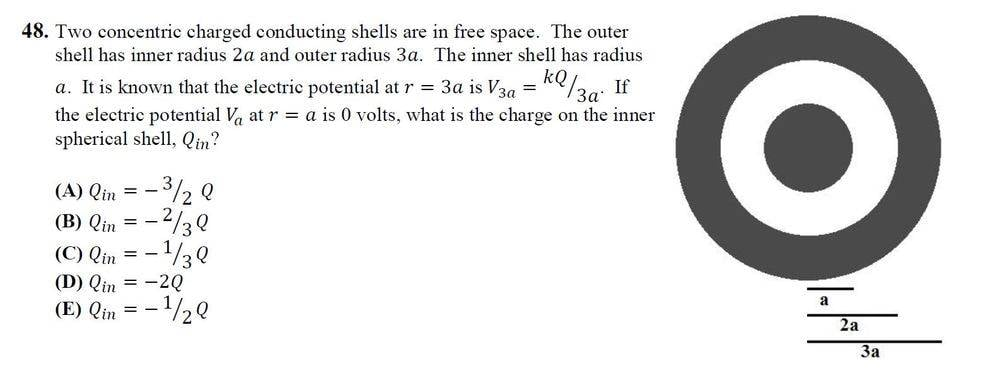# Calculating the charge of two concentric conductive spheres

Miles123K

## Homework StatementThe solution to this problem is B, and I was able to get the answer by calculating the total potential at ##r = 2a##, however, what I don't seem to understand is why must the voltage be calculated at ##r=2a## but not ##r=3a##.

## Homework Equations

##V(r) = - \int_a^b E(r) dr##
##V(r) = \frac 1 {4 \pi \epsilon_0 } \frac Q r##

## The Attempt at a Solution

For solving the problem at ##r=2a##, I used the voltages at ##r=a## and ##r=b##
##V(a) = \frac 1 {4 \pi \epsilon_0 } (\frac {Q_1} {a} + \frac {Q_2} {3a}) = 0## where ##Q_1## is the inner charge and ##Q_2## is the outer charge.
##V(2a) = \frac 1 {4 \pi \epsilon_0 } (\frac {Q_1} {2a} + \frac {Q_2} {3a}) = \frac 1 {4 \pi \epsilon_0 } \frac Q {3a} ##
By simple algebra I was able to solve for ##Q_1## and it was B, however, the condition given was ##V(3a) = \frac 1 {4 \pi \epsilon_0 } \frac Q {3a} ##. why do I have to use the argument that an electrostatic conductor have the same potential throughout to work out the potential ##V(2a)## but not directly solve for ##Q_1## at ##r=3a##?

#### Attachments

Homework Helper
Gold Member
2022 Award
You seem to be ignoring any charge on the inner surface of the outer shell.

•Miles123K
Miles123K
You seem to be ignoring any charge on the inner surface of the outer shell.
Oh right yes. Thanks for the tip. Now I get it. It's actually three equations instead of two.
Here's my solution:
By writing out the potentials and cancelling out the constants, I got the following three equations:
##q_1 + \frac 1 2 q_2 + \frac 1 3 q_3 = 0##
##\frac 1 2 q_1 + \frac 1 2 q_2 + \frac 1 3 q_3 = \frac 1 3 Q##
##\frac 1 3 q_1 + \frac 1 3 q_2 + \frac 1 3 q_3 = \frac 1 3 Q##
By solving this simultaneous equation I got
##q_1 = - \frac 2 3 Q, q_2 = \frac 2 3 Q, q_3 = Q##
##q_1## is the inner most shell, ##q_2## is the inner surface of the outer shell, ##q_3## is the outer surface of the outer shell.

Homework Helper
Gold Member
2022 Award
Oh right yes. Thanks for the tip. Now I get it. It's actually three equations instead of two.
Here's my solution:
By writing out the potentials and cancelling out the constants, I got the following three equations:
##q_1 + \frac 1 2 q_2 + \frac 1 3 q_3 = 0##
##\frac 1 2 q_1 + \frac 1 2 q_2 + \frac 1 3 q_3 = \frac 1 3 Q##
##\frac 1 3 q_1 + \frac 1 3 q_2 + \frac 1 3 q_3 = \frac 1 3 Q##
By solving this simultaneous equation I got
##q_1 = - \frac 2 3 Q, q_2 = \frac 2 3 Q, q_3 = Q##
##q_1## is the inner most shell, ##q_2## is the inner surface of the outer shell, ##q_3## is the outer surface of the outer shell.
Looks right.